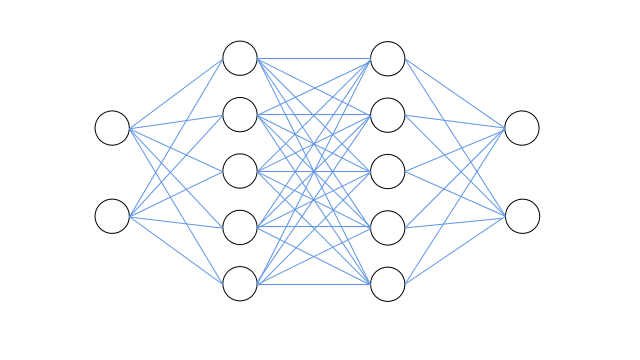# pytorch_第四篇_使用pytorch快速搭建神经网络实现二分类任务（包含示例）

Python 图像处理 OpenCV （16）：图像直方图

# 使用pytorch快速搭建神经网络实现二分类任务（包含示例）

## Introduce

• 利用pytorch已经包装好的库(torch.nn)来快速搭建神经网络结构。
• 利用已经包装好的包含各种优化算法的库(torch.optim)来优化神经网络中的参数，如权值参数w和阈值参数b。

## Build a neural network structure15 张精美动图全面讲解 CORS

• torch.nn.Sequential：是一个时序容器，我们可以通过调用其构造器，将神经网络模块按照输入层到输出层的顺序传入，以此构造完整的神经网络结构，具体用法参考如下神经网络搭建代码。
• torch.nn.Linear：设置网络中的全连接层，用来实现网络中节点输入的线性求和，即实现如下线性变换函数：

\[y = xA^T + b \]

``````
'''

net = nn.Sequential(
nn.Linear(2,5),  # 输入层与第一隐层结点数设置，全连接结构
torch.nn.Sigmoid(),  # 第一隐层激活函数采用sigmoid
nn.Linear(5,5),  # 第一隐层与第二隐层结点数设置，全连接结构
torch.nn.Sigmoid(),  # 第一隐层激活函数采用sigmoid
nn.Linear(5,2),  # 第二隐层与输出层层结点数设置，全连接结构
nn.Softmax(dim=1) # 由于有两个概率输出，因此对其使用Softmax进行概率归一化，dim=1代表行归一化
)

print(net)

'''
Sequential(
(0): Linear(in_features=2, out_features=5, bias=True)
(1): Sigmoid()
(2): Linear(in_features=5, out_features=5, bias=True)
(3): Sigmoid()
(4): Linear(in_features=5, out_features=2, bias=True)
(5): Softmax(dim=1)
)'''

``````

## Configure Loss Function and Optimizer

note: torch.optim库中封装了许多常用的优化方法，这边使用了最常用的随机梯度下降来优化网络参数。例子中使用了交叉熵损失作为代价函数，其实torch.nn中也封装了许多代价函数，具体可以查看官方文档。对于pytorch中各种损失函数的学习以及优化方法的学习将在后期进行补充。

``````# 配置损失函数和优化器
optimizer = torch.optim.SGD(net.parameters(),lr=0.01) # 优化器使用随机梯度下降，传入网络参数和学习率
loss_func = torch.nn.CrossEntropyLoss() # 损失函数使用交叉熵损失函数
``````

## Model Training

``````# 模型训练
num_epoch = 10000 # 最大迭代更新次数
for epoch in range(num_epoch):
y_p = net(x_t)  # 喂数据并前向传播

loss = loss_func(y_p,y_t.long()) # 计算损失
'''
PyTorch默认会对梯度进行累加，因此为了不使得之前计算的梯度影响到当前计算，需要手动清除梯度。
pyTorch这样子设置也有许多好处，但是由于个人能力，还没完全弄懂。
'''
loss.backward()  # 计算梯度，误差回传
optimizer.step()  # 根据计算的梯度，更新网络中的参数

if epoch % 1000 == 0:
print('epoch: {}, loss: {}'.format(epoch, loss.data.item()))

'''

epoch: 0, loss: 0.7303197979927063
epoch: 1000, loss: 0.669952392578125
epoch: 2000, loss: 0.6142827868461609
epoch: 3000, loss: 0.5110923051834106
epoch: 4000, loss: 0.4233965575695038
epoch: 5000, loss: 0.37978556752204895
epoch: 6000, loss: 0.3588798940181732
epoch: 7000, loss: 0.3476340174674988
......
'''

print("所有样本的预测标签: \n",torch.max(y_p,dim = 1))

'''
note：可以发现前100个标签预测为0，后100个样本标签预测为1。因此所训练模型可以正确预测训练集标签。

tensor([0, 0, 0, 0, 0, 0, 0, 0, 0, 0, 0, 0, 0, 0, 0, 0, 0, 0, 0, 0, 0, 0, 0, 0,
0, 0, 0, 0, 0, 0, 0, 0, 0, 0, 0, 0, 0, 0, 0, 0, 0, 0, 0, 0, 0, 0, 0, 0,
0, 0, 0, 0, 0, 0, 0, 0, 0, 0, 0, 0, 0, 0, 0, 0, 0, 0, 0, 0, 0, 0, 0, 0,
0, 0, 0, 0, 0, 0, 0, 0, 0, 0, 0, 0, 0, 0, 0, 0, 0, 0, 0, 0, 0, 0, 0, 0,
0, 0, 0, 0, 1, 1, 1, 1, 1, 1, 1, 1, 1, 1, 1, 1, 1, 1, 1, 1, 1, 1, 1, 1,
1, 1, 1, 1, 1, 1, 1, 1, 1, 1, 1, 1, 1, 1, 1, 1, 1, 1, 1, 1, 1, 1, 1, 1,
1, 1, 1, 1, 1, 1, 1, 1, 1, 1, 1, 1, 1, 1, 1, 1, 1, 1, 1, 1, 1, 1, 1, 1,
1, 1, 1, 1, 1, 1, 1, 1, 1, 1, 1, 1, 1, 1, 1, 1, 1, 1, 1, 1, 1, 1, 1, 1,
1, 1, 1, 1, 1, 1, 1, 1])
'''

``````

## 网络的保存和提取

``````'''两种保存方式

torch.save(net,'net.pkl')

torch.save(net.state_dict(),'net_parameter.pkl')

加载时需要提供两个信息:
第一: 网络结构信息，需要先重新搭建和保存的网络同样的网络结构。
第二: 保存的网络中的参数的信息，权值和阈值参数。
具体加载方式如下:
net = nn.Sequential(
nn.Linear(2,5),
torch.nn.Sigmoid(),
nn.Linear(5,5),
torch.nn.Sigmoid(),
nn.Linear(5,2),
nn.Softmax(dim=1)
)
'''

``````

## 附完整代码

``````import torch
import torch.nn as nn

'''

torch.normal(tensor1,tensor2)

torch.normal以此抽取tensor1和tensor2中对应位置的元素值构造对应的正态分布以随机生成数据，返回数据张量。
'''

x1_t = torch.normal(2*torch.ones(100,2),1)
y1_t = torch.zeros(100)

x2_t = torch.normal(-2*torch.ones(100,2),1)
y2_t = torch.ones(100)

x_t = torch.cat((x1_t,x2_t),0)
y_t = torch.cat((y1_t,y2_t),0)

'''

'''

net = nn.Sequential(
nn.Linear(2,5),  # 输入层与第一隐层结点数设置，全连接结构
torch.nn.Sigmoid(),  # 第一隐层激活函数采用sigmoid
nn.Linear(5,5),  # 第一隐层与第二隐层结点数设置，全连接结构
torch.nn.Sigmoid(),  # 第一隐层激活函数采用sigmoid
nn.Linear(5,2),  # 第二隐层与输出层层结点数设置，全连接结构
nn.Softmax(dim=1) # 由于有两个概率输出，因此对其使用Softmax进行概率归一化
)

print(net)
'''
Sequential(
(0): Linear(in_features=2, out_features=5, bias=True)
(1): Sigmoid()
(2): Linear(in_features=5, out_features=5, bias=True)
(3): Sigmoid()
(4): Linear(in_features=5, out_features=2, bias=True)
(5): Softmax(dim=1)
)'''

# 配置损失函数和优化器
optimizer = torch.optim.SGD(net.parameters(),lr=0.01) # 优化器使用随机梯度下降，传入网络参数和学习率
loss_func = torch.nn.CrossEntropyLoss() # 损失函数使用交叉熵损失函数

# 模型训练
num_epoch = 10000 # 最大迭代更新次数
for epoch in range(num_epoch):
y_p = net(x_t)  # 喂数据并前向传播

loss = loss_func(y_p,y_t.long()) # 计算损失
'''
PyTorch默认会对梯度进行累加，因此为了不使得之前计算的梯度影响到当前计算，需要手动清除梯度。
pyTorch这样子设置也有许多好处，但是由于个人能力，还没完全弄懂。
'''
loss.backward()  # 计算梯度，误差回传
optimizer.step()  # 根据计算的梯度，更新网络中的参数

if epoch % 1000 == 0:
print('epoch: {}, loss: {}'.format(epoch, loss.data.item()))

'''
torch.max(y_p,dim = 1)是每行最大的值
torch.max(y_p,dim = 1)是每行最大的值的下标，可认为标签
'''
print("所有样本的预测标签: \n",torch.max(y_p,dim = 1))

``````

pytorch_第四篇_使用pytorch快速搭建神经网络实现二分类任务（包含示例）

three.js 着色器材质之初识着色器